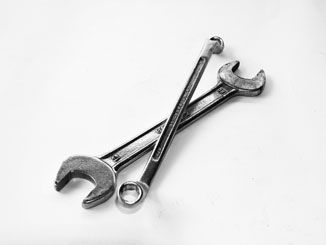# Generating a union of overlapping intervals

This function takes a set of overlapping intervals and returns the union of the intervals.
r code-snippet
Published

April 10, 2018

This function takes a set of overlapping intervals and returns the union of the intervals.

## Use case example

• calculate the total duration a patient has had any line in place
• one row per line
• columns of start and end times for each line
• if two lines are in place at the same time, they should not be double-counted to contributing to duration
• so, you need to generate a non-overlapping set of intervals prior to calculating the total duration
``````########## Function to generate non-overlapping intervals ##########
# - dataframe with row of intervals, with column names 'start' and 'stop'
# - consolidates overlapping intervals and returns a dataframe with non-redundant, non-overlapping dataframes
# - logic of the if(elseif) -> if: works but might not be optimized

library(dplyr)                                         # for %>%, arrange, bind_rows

interval_union <- function(input) {
if (nrow(input) == 1) {                              # if only 1 input interval, return it
return(input)
}
input <- input %>% arrange(start)                    # sort inputs by start
output = input[1, ]                                  # start off output with just the first (earliest) interval
for (i in 2:nrow(input)) {                           # loop from 2nd to last input interval
x <- input[i, ]                                    # the next interval to work on

if (output\$stop[nrow(output)] < x\$start) {         # the next interval starts after the end of the last output interval...
output <- bind_rows(output, x)                   # ... so add it as a NEW output interval; it will not be the last output interval
} else if (output\$stop[nrow(output)] == x\$start) { # the next interval starts exactly at the end of the last output interval...
output\$stop[nrow(output)] <- x\$stop              # ... so just extend the previous last output interval; it's still the last one
}
if (x\$stop > output\$stop[nrow(output)]) {          # the next interval ENDS after the end of the last output interval...
output\$stop[nrow(output)] <- x\$stop              # ... so just extend the previous last output interval; it's still the last one
}
}
return(output)
}``````
``````d <- data.frame(
start = c('2005-01-01', '2000-01-01', '2001-01-01'),
stop = c('2006-01-02', '2001-01-02', '2004-01-02'),
stringsAsFactors = FALSE
)
d``````
``````       start       stop
1 2005-01-01 2006-01-02
2 2000-01-01 2001-01-02
3 2001-01-01 2004-01-02``````
``interval_union(d)``
``````       start       stop
1 2000-01-01 2004-01-02
2 2005-01-01 2006-01-02``````
Note

It would definitely be possible to rewrite this using just base R, but because all my coding projects are in the tidyverse, I prefer to continue using its functions.